Courses
Courses for Kids
Free study material
Offline Centres
More

# X-Axis and Y-AxisLast updated date: 27th Nov 2023
Total views: 103.5k
Views today: 2.03k## An Introduction to an X-axis and Y-axis

The x-axis and y-axis are two important lines that make a graph. A graph consists of a horizontal axis and a vertical axis where data can be represented. There are two ways to describe a point, horizontally and vertically, which are easily understood using a graph. In a graph, the x-axis is horizontal and the y-axis is vertical. In this article, we will learn about the x-axis and y-axis and we will also see the graph plotter with points along with solving a few examples.

## X-axis and Y-axis

Any point on the coordinate plane can be described by an ordered pair, which is written as (x-coordinate, y-coordinate) where the x-coordinate denotes a point on the x-axis and the y-coordinate denotes a point on the y-axis. The ordinate or "y-axis" is another name for the y-axis and another name for the x-axis is known as the "abscissa" or "x graph."

## X-axis and Y-axis on the Graph

A graph is made up of two crucial lines called the x and y axes. In a graph, data can be represented on both the horizontal and vertical axes. A graph makes it simple to understand how a point might be defined vertically or horizontally. The x-axis and y-axis on the graph are these two horizontal and vertical lines or axes, respectively. As the origin is where the x- and y-axes intersect.X-axis and Y-axis Graph

## Any Point on the Y-axis Is of the Form

The fact that each point on the graph (straight line) of a linear equation involving two variables corresponds to a solution to the linear equation. As a result, each answer to the linear equation can be represented as a distinct point on the equation's graph. Lines parallel to the y-axis and the x-axis, respectively, represent the graphs of x = a and y = a.

The shape of any point on the y-axis is of the form (0, y).

## The Ordinate of All Points on the X-axis

Any point on the x-axis has coordinates of (x,0), where x is the abscissa and 0 is the ordinate. As a result, each point on the x-axis has an ordinate of 0. Thus, the ordinate of all points on the x-axis is zero.

## Graph Plotter with Points

Take the linear equation y = 2x+1 as an example. Now, create a table with two columns for the values of x and y to graph this equation then draw a graph as follows:

Now by substituting the values in one of the variables we will complete the table.

So, if when x = 0

y = 2(0) + 1

=1

Similarly, when x = 1, y = 3 and when x = 2, y = 5.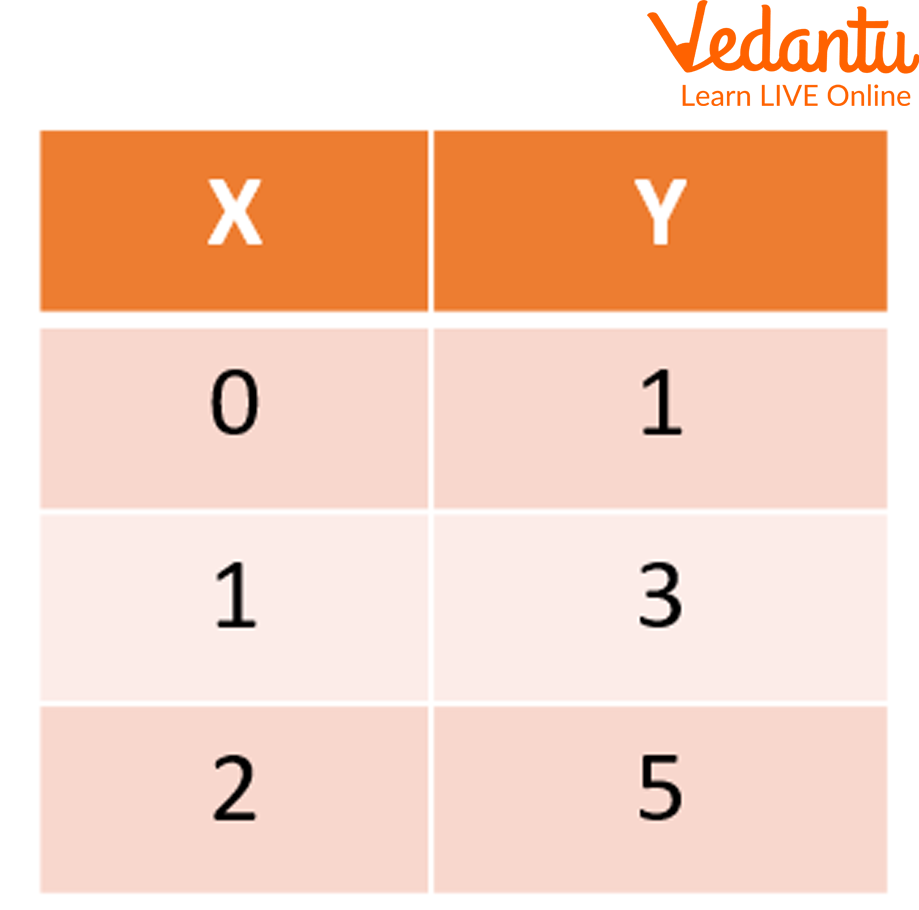Graph Plotter

Now, we will plot the graph based on the above table.

• So for locating the points on the graph, we just have to point to the x and y-axis.

• So if x = 0 and y = 1. For this, the x-axis will be zero and on the y-axis, we have to go up to 1.

• Therefore, the point will be (0,1). Similarly, other points can be drawn. In the end, join these points.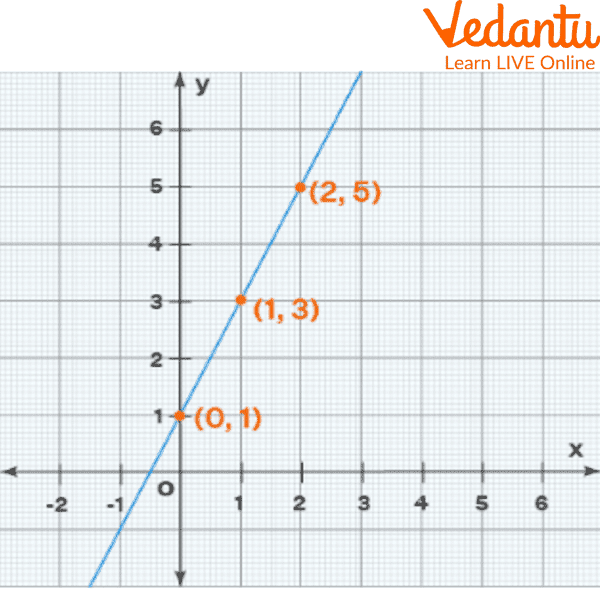Graph Using Plotter Points

## Solved Examples

Q 1. The table for the X and Y graph shows the population of a city from 2015 to 2020 as: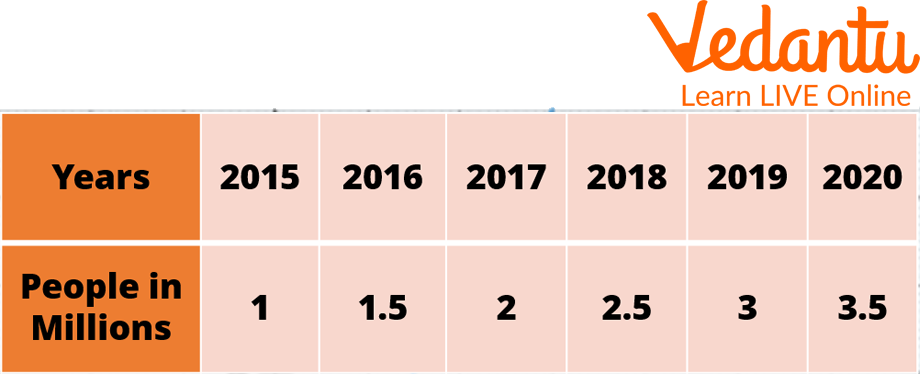Graph Plotter (Example 1)

Ans: The ordered pair is written as (x-coordinate, y-coordinate) or (x, y), where the x and y-coordinate implies a point on the x-axis or perpendicular distance from the y-axis whereas another one implies a point on the y-axis or perpendicular distance from the x-axis respectively. Each point is located on the graph indicated by an ordered pair with the x-axis coming first and the y-axis coming second. The chart is as follows: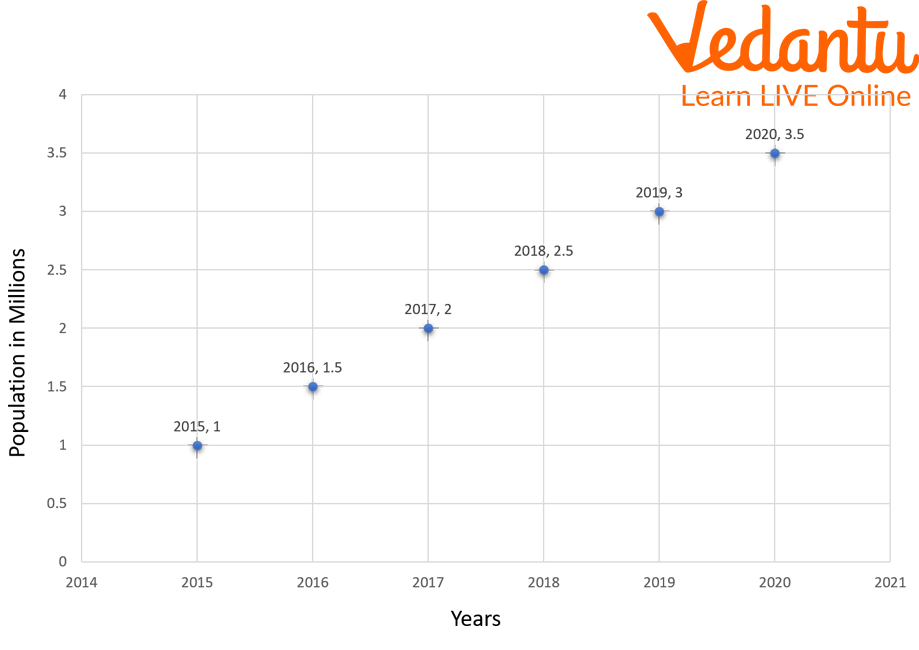Graph (Example 1)

Q 2. Daniel's teacher assigns him a maths task with x and y axes that requires him to graph the points (3, 2) and (2, 3) and draw a line that connects them. Can you point to the location where it intersects the x-axis?

Ans: The graph can be used to plot the points as displayed.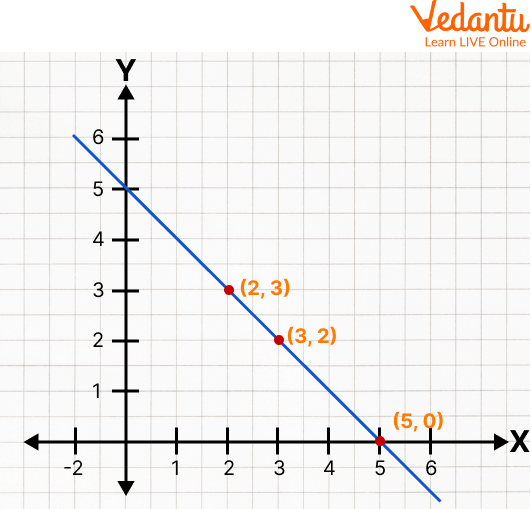Graph

Consequently, the line crosses the x-axis at that location (5, 0).

## Practice Questions

Q 1. Draw a coordinate system and place the coordinates (1,2), (0,4), and (-1,-3) on it. Are all of the points on a line? Can you name the line?

Q 2. State true or false.

1. On the y-axis, the x coordinate is nonzero and the y coordinate is zero. Ans: False

2. The x-axis is a vertical line. Ans: False

3. On the x-axis, the x coordinate is nonzero and the y coordinate is zero. Ans: True

4. The y-axis is a vertical line. Ans: True

## Summary

Any point on the coordinate plane is well defined by an ordered pair where the ordered pair is written as (x-coordinate, y-coordinate) or (x,y), where the x-coordinate represents a point on the x-axis or perpendicular distance from the y-axis and the y-coordinate represents a point on the y-axis or perpendicular distance from the x-axis. The point where the x-axis and y-axis intersect is known as the origin and is used as the reference point for the plane. The x-axis is also known as abscissa or x graph whereas the y-axis is also known as ordinate or y graph. Later, we have also seen how to plot the graph by using linear equations. In the end, we have included, solved examples, and practised problems to get a better understanding of the topic.

## FAQs on X-Axis and Y-Axis

1. Which point is on the negative y-axis?

The point which has a negative value of its y-coordinate is on the negative y-axis.

2. What are the 4 quadrants in a graph?

• Quadrant 1: Is the positive side of both the x and y-axis.

• Quadrant 2: Are the negative side of the x-axis and the positive side of the y-axis.

• Quadrant 3: Is the negative side of both the x and y-axis.

• Quadrant 4: Are the negative side of the y-axis and the positive side of the x-axis.

3. How should an axis graph be read?

The horizontal or x-axis is the line along the bottom, and the vertical or y-axis is the line up the side. Both categories and numerals are possible on the x-axis. Starting at the bottom left of the graph, you read it. Numbers are typically displayed on the y-axis, again beginning at the bottom left of the graph. The y-axis numbers typically, but not always, begin at 0 in the graph's lower left corner and proceed upward.

y-axis should contain the dependent variable (vertical line). At the origin, where the coordinates are, the x and y axes intersect (0,0).